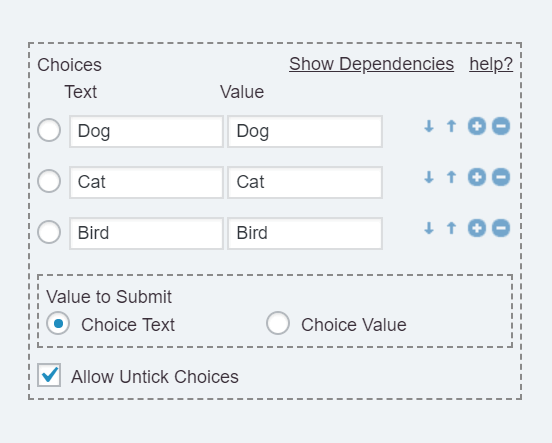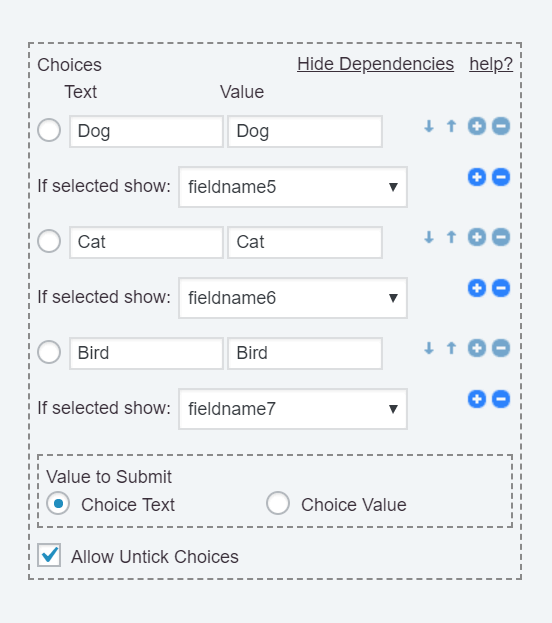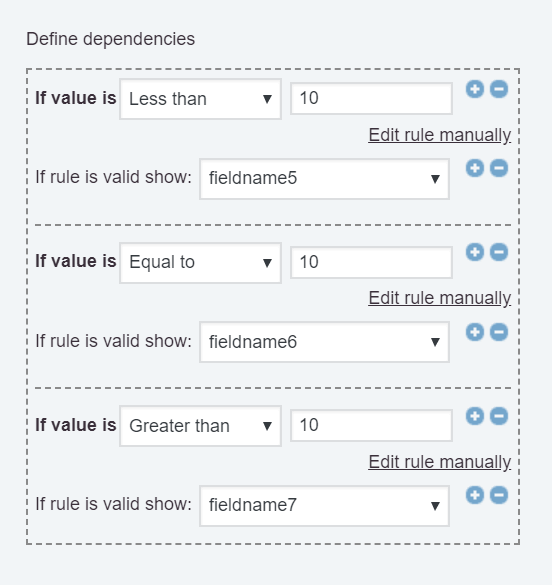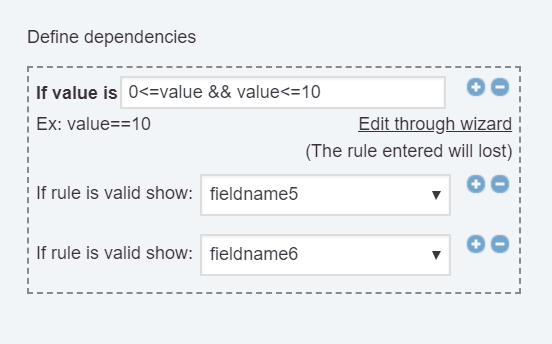# Calculated Fields Form Blog

Tips and cases of use for a successful WordPress website with calculated forms.

Blog / Dependencies between fields

## Dependencies between fields

There are many cases of use in which some fields depend on the values of other fields in the form, for example, in e-commerce with different types of products, a lodging project with different rooms typologies, etc.

Our plugin allows defining dependencies in the following controls: DropDown, Radio Buttons, Checkbox, and the Calculated Fields.

#### DropDown, Radio Buttons, and Checkbox fields

For DropDown, Radio Buttons and Checkbox fields the behavior is similar. All of them include the choices section:Click on the "Show Dependencies" link in the choices section, to display the dependency controls and dependent fields lists:Below every choice would be included a list with the fields in the form. Select the field dependent on the corresponding choice. To define multiple fields dependent on the same choice, click on the plus (+) icon, to display another list of fields, and select the new field from the list, and so on. To remove a dependency, press the minus (-) icon.

The dependent fields would be visible and active, only if it is ticked or selected the choice where they are defined:Pay attention: The dependent fields would be sent to the server when the form is submitted only if they are active.

The values of dependent fields are zero when they are inactive.

#### Calculated Fields Form

The calculated fields include a specific section where to define the dependencies, but in this case, the other fields depend on the equations' results.

To define dependencies in the calculated fields:

1. Select the calculated field in the form.
2. Through the "Define dependencies" section, select the comparison rule from "if the value is" list (the alternatives are: Equal to, Not equal to, Greater than, Greater than or equal to, Less than, Less than or equal to), and enter the value for comparing through the input box.
3. Select the field to display if the rule is valid.

Note: Pressing the "plus" (+) button beside the list of dependent fields it is possible to apply the same dependency rule to multiple fields.

Note 2: Pressing the "plus" (+) button beside the dependency rule it is possible to define additional dependency rules.For complex dependencies, click on the Edit rule manually link, and enter the dependency rule manually. The value word in the rule's definition is a reference to the equation's result. In the screenshot below the fields: fieldname5 and fieldname6 become active if the equation's result is between 0 and 10, both included:#### How to define dependencies with other fields?

The plugin does not allow create dependencies in other controls, however, there is possible to use calculated fields as auxiliary to create them. Simply, use the field as part of the equation associated with the calculated field, and define the dependency rules based on the equation's result like in the previous section.

Note: The auxiliary calculated fields are not relevant in the form's interface, to hide them, tick the checkbox: "Hide Field From Public Page" in their settings.

For example: in an e-commerce form, where the fields for financing the purchase should be displayed if the amount entered by the user through the currency field: fieldname1 is greater than \$1000.

1. Insert a calculated field in the form with the following equation: fieldname1
2. And then, define the dependency as follows: If the value is greater than 1000 display the fields required for financing the purchase.

#### How to define dependencies depending on the values of multiple fields at once?

Similar to the previous section, it is required to use calculated fields as auxiliary.

For example, assuming you want to display the fieldname3 field if the choice selected through the fieldname1 is 10, and the value entered through the fieldname2 is greater than 5:

1. Insert a calculated field in the form with the following equation piece of code as its equation:

``````IF(AND(fieldname1 == 10, 5<fieldname2), 1, 0)
``````
2. Now, define the dependency as follows, if the value is equal to 1, select the fieldname3 from the list of dependent fields.